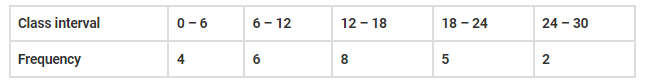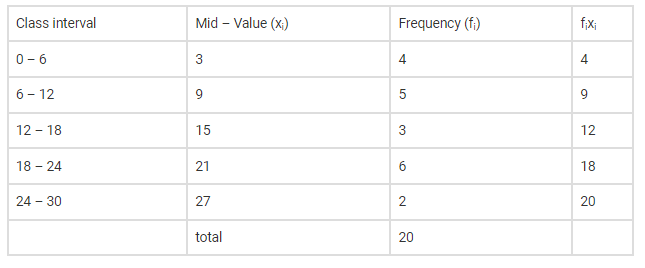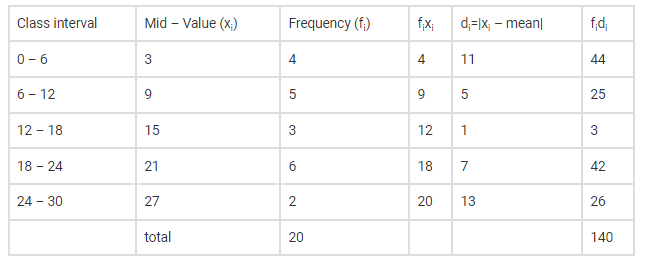Deepak Scored 45->99%ile with Bounce Back Crack Course. You can do it too!

# Calculate the mean deviation from the median of the following data:

Question:

Calculate the mean deviation from the median of the following data:Solution:

Given the frequency distribution

Now we have to find the mean deviation from the median

Let us make a table of the given data and append other columns after calculationsNow, here N=20, which is even.

Now, here $\mathrm{N}=20$, which is even.

Here median class $=\frac{\mathrm{N}}{2}=10^{\text {th }}$ term

This observation lie in the class interval 12-18, so median can be written as,

$\mathrm{M}=\mathrm{l}+\frac{\frac{\mathrm{N}}{2}-\mathrm{cf}}{\mathrm{f}} \times \mathrm{h}$

Here $I=12, c f=9, f=3, h=6$ and $N=20$, substituting these values, the above  equation becomes,

$M=12+\frac{\frac{20}{2}-9}{3} \times 6$

$\Rightarrow M=12+\frac{10-9}{3} \times 6$

$\Rightarrow \mathrm{M}=12+\frac{1 \times 6}{3}$

$\Rightarrow M=12+2=14$Hence Mean Deviation becomes,

$M . D=\frac{\sum f_{i} d_{i}}{\sum f_{i}}=\frac{140}{20}=7$

Therefore, the mean deviation about the median of the distribution is 7